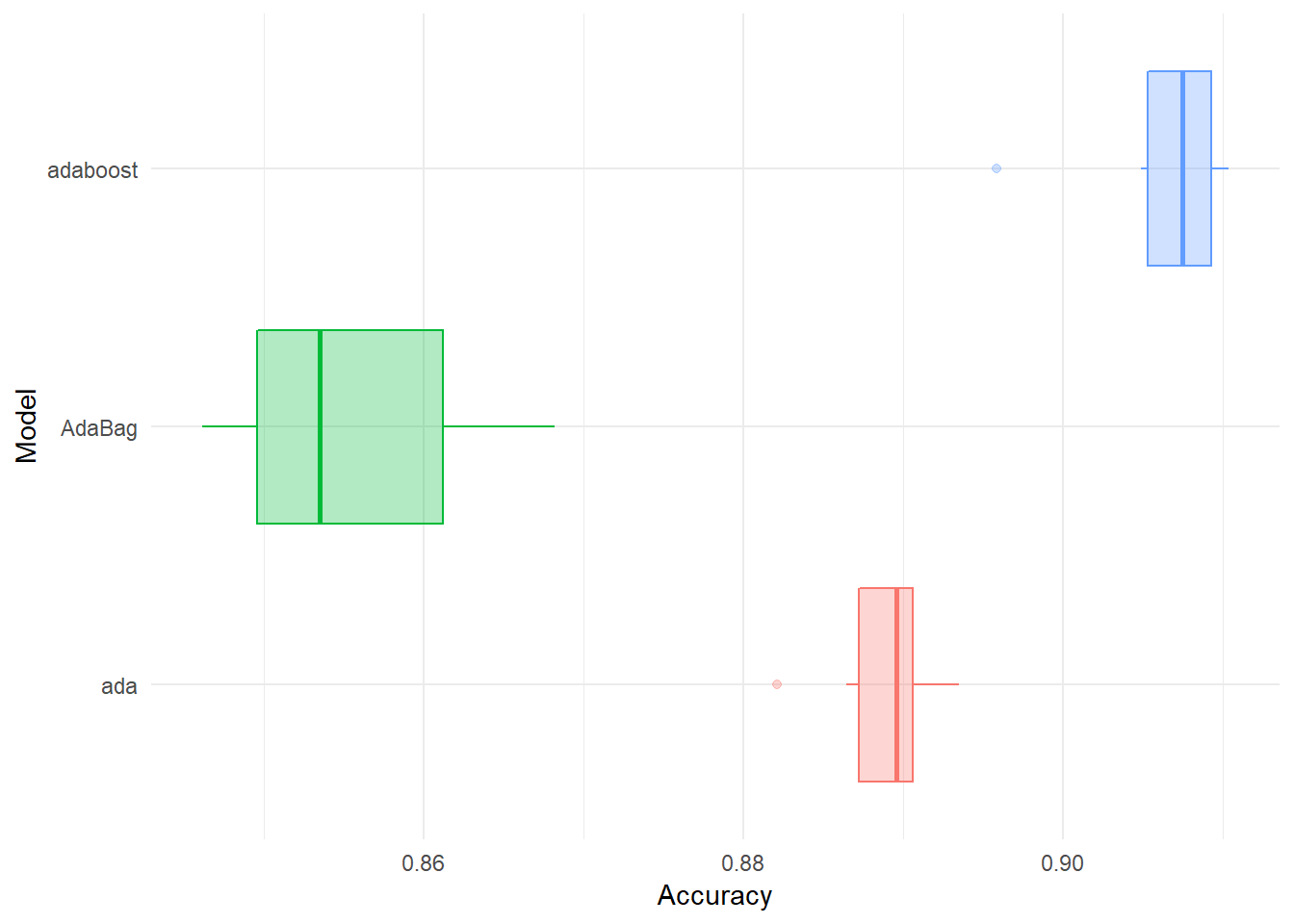# Introduction

In this post, I will present the way of training all 182 Machine Learning Models for Classification. It would be better for data scientists to pick up one model with the best performance out of all possible models objectively, rather than subjectively choose the one that is frequently referred.

# Remove workspace if needed:
# rm(list = ls())

library(caret)

# Get list of all models:
all_model <- modelLookup()

library(tidyverse)
library(magrittr)

# Process

## Stage 1: Install all packages needed

# Get all models for classification:

classification_model <- all_model %>%
filter(forClass == TRUE, !duplicated(model))

# All packages will be used for training these models:

all_packages <- sapply(classification_model$model, function(x) { x %>% getModelInfo() %>% .[] %>% .[["library"]]}) %>% unlist() all_packages <- all_packages[!duplicated(all_packages)] # All R package had being installed on your computer: your_packages <- installed.packages() %>% as.data.frame() %>% pull(Package) %>% as.character() # Some packages that can not be installed from CRAN: cannot_installed <- c("adaptDA", "CHAID", "sparsediscrim", "elmNN", "gpls", "logicFS", "FCNN4R", "mxnet", "vbmp") # Coresponding LM approaches: ml_not_used <- c("amdai", "chaid", "dda", "elm", "gpls", "logicBag", "mlpSGD1", "mxnet", "vbmpRadial") # Packages must be installed: additional_package <- all_packages[!all_packages %in% your_packages] additional_package <- all_packages[!all_packages %in% cannot_installed] # Number of packages will be installed: additional_package %>% length() ##  86 Now it’s time to install all packages. I already had all these packages in library then I will skip this step. # install.packages(pkgs = additional_package, dependencies = TRUE) # Thus, some ML Models should use: should_use_ml <- classification_model %>% filter(!model %in% ml_not_used) ## Stage 2: Train 5 ML Models # List of all models can train by using caret package: all_model <- should_use_ml$model

# Import data:
hmeq <- read.csv("http://www.creditriskanalytics.net/uploads/1/9/5/1/19511601/hmeq.csv")
#------------------------------------
# Perform some data pre-processings
#------------------------------------

# Function replaces NA by mean:
replace_by_mean <- function(x) {
x[is.na(x)] <- mean(x, na.rm = TRUE)
return(x)
}

# A function imputes NA observations for categorical variables:

replace_na_categorical <- function(x) {
x %>%
table() %>%
as.data.frame() %>%
arrange(-Freq) ->> my_df

n_obs <- sum(my_df$Freq) pop <- my_df$. %>% as.character()
set.seed(29)
x[is.na(x)] <- sample(pop, sum(is.na(x)), replace = TRUE, prob = my_df$Freq) return(x) } # Use the two functions: df <- hmeq %>% mutate_if(is.factor, as.character) %>% mutate(REASON = case_when(REASON == "" ~ NA_character_, TRUE ~ REASON), JOB = case_when(JOB == "" ~ NA_character_, TRUE ~ JOB)) %>% mutate_if(is_character, as.factor) %>% mutate_if(is.numeric, replace_by_mean) %>% mutate_if(is.factor, replace_na_categorical) # Convert BAD to factor and scale the dataset into a range of [0;1]: df_for_ml <- df %>% mutate(BAD = case_when(BAD == 1 ~ "Bad", TRUE ~ "Good") %>% as.factor()) %>% mutate_if(is.numeric, function(x) {(x - min(x)) / (max(x) - min(x))}) #----------------------------------- # Simultaneously Train 3 Models #----------------------------------- # Split data: set.seed(1) id <- createDataPartition(y = df_for_ml$BAD, p = 0.7, list = FALSE)
df_train_ml <- df_for_ml[id, ]
df_test_ml <- df_for_ml[-id, ]

# Set conditions for training model and cross-validation:

set.seed(1)
number <- 3
repeats <- 2

control <- trainControl(method = "repeatedcv",
number = number ,
repeats = repeats,
classProbs = TRUE,
savePredictions = "final",
index = createResample(df_train_ml$BAD, repeats*number), summaryFunction = multiClassSummary, allowParallel = TRUE) # Use Parallel computing: library(doParallel) registerDoParallel(cores = detectCores() - 1) # Simultaneously train some machine learning models: library(caretEnsemble) set.seed(1) # List all models that you want to train. For purpose of explanation # I will only use 5 models: my_models <- all_model[1:3] In case my laptop just has 8 cores and 16 GB, I will be running 3 models. If you have a more powerful laptop or a cloud account, you can run all model. # Train these ML Models: model_list1 <- caretList(BAD ~., data = df_train_ml, trControl = control, metric = "Accuracy", methodList = my_models) # Extract all results fromML ML models: list_of_results <- lapply(my_models, function(x) {model_list1[[x]]$resample})

# Convert to data frame:
df_results <- do.call("bind_rows", list_of_results)

df_results %<>% mutate(Model = lapply(my_models, function(x) {rep(x, number*repeats)}) %>% unlist())
# Comparing among models based on a criteria of model performance selected:

df_results %>%
select(Accuracy, Model) %>%
ggplot(aes(Model, Accuracy, fill = Model, color = Model)) +
geom_boxplot(show.legend = FALSE, alpha = 0.3) +
theme_minimal() +
coord_flip()# Or use some statistics for comparing:

df_results %>%
select(Accuracy, Model) %>%
group_by(Model) %>%
summarise_each(funs(min, max, median, mean, sd, n()), Accuracy) %>%
arrange(-mean) %>%
mutate_if(is.numeric, function(x) {round(x, 3)}) %>%
knitr::kable()
Model min max median mean sd n
adaboost 0.896 0.910 0.907 0.906 0.005 6
ada 0.882 0.894 0.890 0.889 0.004 6
AdaBag 0.846 0.868 0.853 0.856 0.009 6